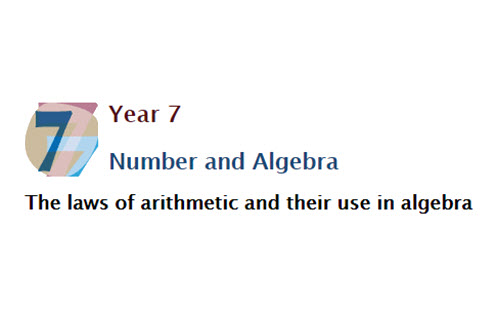Go to website

# Laws of arithmetic

This resource guides and supports the teacher to explicitly teach the laws and properties of arithmetic using algebraic terms and expressions to model examples. Students can use the resource to guide their understanding to identify order of operations in contextualised problems, preserving the order by inserting brackets in numerical expressions, then recognising how order is preserved by convention. They can follow through the examples presented and complete practice questions.

Year level(s) Year 7
Audience Student, Teacher
Purpose Content knowledge, Teaching strategies, Evidence-based approaches, Student task
Teaching strategies and pedagogical approaches Explicit teaching, Worked examples, Collaborative learning
Keywords algebra, cummulative law, assosciative law, distributive law, order of operation, powers, notation, explicit teaching

## Curriculum alignment

Curriculum connections English, Numeracy, Critical and creative thinking
Strand and focus Algebra, Number, Apply understanding, Build understanding
Topics Addition and subtraction, Algebraic expressions, Patterns and algebra, Operating with number, Multiples, factors and powers, Multiplication and division
AC: Mathematics (V9.0) content descriptions
AC9M7A02
Formulate algebraic expressions using constants, variables, operations and brackets

Numeracy progression Number patterns and algebraic thinking (P6)